# Two Plane Balancing Example with DAQmx

Publish Date: Mar 08, 2016 | 11 Ratings | 4.27 out of 5 | Print | 3 Customer Reviews | Submit your review

## Overview

Unbalance is a very common source of high vibration that is identified by excessive levels of vibration amplitudes at a frequency that is synchronous with machine speed. Unbalance happens because the densities of materials are uneven; shafts are not perfectly round or not mounted exactly in the center, etc. Weight unbalance in a rotating system often produces excessive synchronous forces that reduce the life spans of various mechanical elements. Therefore, balancing is important in the manufacture and maintenance process of rotating machines.

### 1. Introduction

Balancing usually is performed in two ways -- shop balancing and field balancing. Shop balancing mounts the mechanical parts on a balancing machine and balances each part separately. Field balancing balances the machine in normal operations with the rotor mounted in the bearings. Large machines, such as steam turbines, electric motors, and generator armatures, often require field balancing. Field balancing times average 4 hours and rates range from \$60 to \$200 per hour. USB dynamic signal acquisition (DSA) devices coupled with a laptop make for an ideal field balancing system because they are portable and lightweight.

This two-plane balancing demo is built with LabVIEW and DAQmx to be used with a USB-9234 or other DAQmx based dynamic signal acquisition card. This demo shows how to use the device to measure the vibrations of rotating machinery and reduce the vibrations through balancing. You can use the demo VIs in real-world applications with some simple modifications to the code.

Note that this code is built on the Sound and Vibration Measurement Suite, which is required to run it.  You may obtain a free 7 day trial if you wish here.

Two Plane Balancing Example

### 3. Fundamentals of Two Plane Balancing

There are usually two types of unbalance: static unbalance and dynamic unbalance.Figure 1.

As shown in Figure1, static unbalance happens when the gravity centre of a rotor shift from the geometrical center of the thin plane. In some cases, you can place the rotor on knife edges, and allow gravity to pull the heavy spot down to the bottom of the assembly to detect static unbalance. The thin planes in real cases can be fans, grinding discs, pulleys, flywheels, gears and so on. They share a common characteristic that the rotors are quite narrow and have no axial swash motion. You can remove static unbalance by adding/removing balancing screws (or sliding weights) or drilling/grinding on the rotor elements.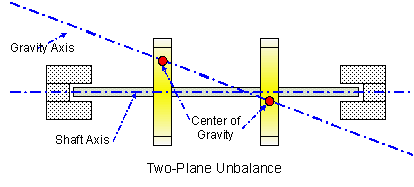Figure 2.

As shown in Figure2, the next level of unbalance complexity consists of weight mal-distribution in two separate geometric planes. You need to establish two-plane balancing when the rotors are elongated and rigid, such as electric motors, generator armatures, machine spindles, grinding rolls and so on.

Correction of unbalance situations involves characterizing the heavy spot. The heavy spot is the radial location at which the excessive radial mass distribution exists. This heavy spot is always a location that is opposite the location where weight needs to be added. Unfortunately, unless previous balancing information is available, the location of the heavy spot cannot be identified directly. In this case, you can use the Influence Coefficient method for calculation of correction weights as many field balancing solutions do.

The influence coefficient is used to describe how the rotor system responds to the unbalanced weight changes. Once a rotor installs a trial weight to produce a change in vibration amplitude or phase, the influence caused by the trial weight, or the influence coefficients can be calculated. A single-plane balance procedure will produce one balance response coefficient. Multiple-plane balancing will produce a number of coefficients depending upon the number of balance planes. Since the initial vibration can be viewed as the response of the rotor to the initial mass unbalance, you can calculate the initial mass unbalance with the initial vibration and the influence coefficients.

The system shown in Figure3 is a typical two-plane balancing system. The whole system consists of a rotor, two bearings, two accelerometers and one optical tachometer. The accelerometers are mounted on the bearings and used to acquire vibration signals. The tachometer signal is used to calculate the rotating speed and synchronize the vibration signals in each revolution.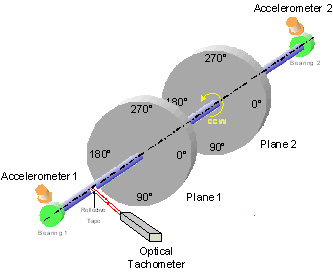Figure 3.

Assuming the rotor system is a linear system, the response at each measurement point (the bearing point) is equal to the vector summation of the unbalance response at each plane. This means the weight at plane 1 can contribute to the vibration acquired at bearing 1 as well as the vibration acquired at bearing 2. So does the weight at plane 2. The basic relationship between the vibration response and the unbalance weight at the planes can commonly be expressed by a group of sensitivity vectors. By adding a known calibration weight at a known angular location on the planes, and measuring the vibration response vectors at both bearings, you can determine the sensitivity vectors experimentally. Once you figure out the sensitivity vectors, you can calculate the initial unbalance weight at each plane and make corrections. The details of this method are introduced in the appendix. You can refer to the appendix if you want to know more about this method.

### 4. Hardware Setup

The mechanical connections are shown below in Figure 4. The accelerometers are powered by the internal excitation of the DSA card.  The tachometer should be a 0-5 TTL pulse that provides rotation information about the system.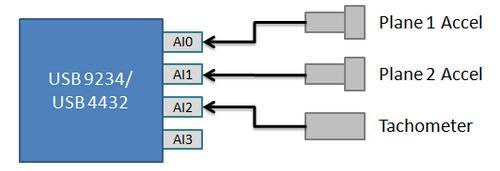Figure 4.

### 5. Using the Software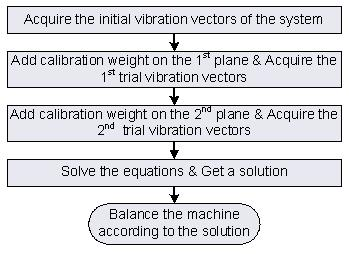Figure 5.

The process of the demo is illustrated in Figure 5 above.  Before running the software you must first configure the software to run properly on the Configuration Tab as shown below in Figure 6.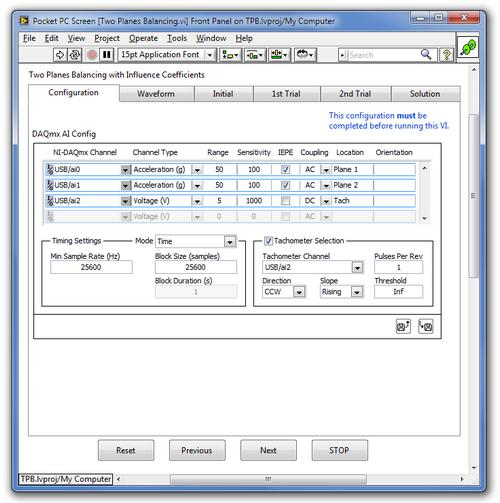Figure 6. The Configuration Tab

You can then being to run the VI and move over to the Waveform tab.  On this tab you can verify the proper signals are being acquired from the tachometer and two accelerometers by pressing the Acquire button.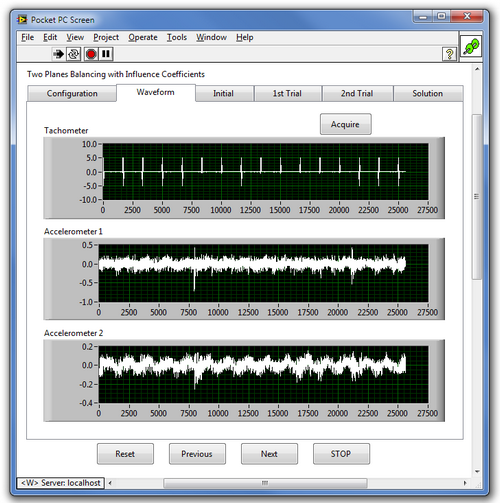Figure 7. Initial acquisition of waveforms.

Once the signals have been verified you can move the the Initial tab to being balancing.  This tab allows you to acquire the initial vibrations of the system.  You need to set the the number of acquisition to average before taking your initial acquisition.  This value will be carried through the process.  Keep in mind that taking a higher number of averages improves accuracy, but also will increase the time required for balancing.  Once you are satisfied with the number of averages, press the Acquire button again to record your initial values.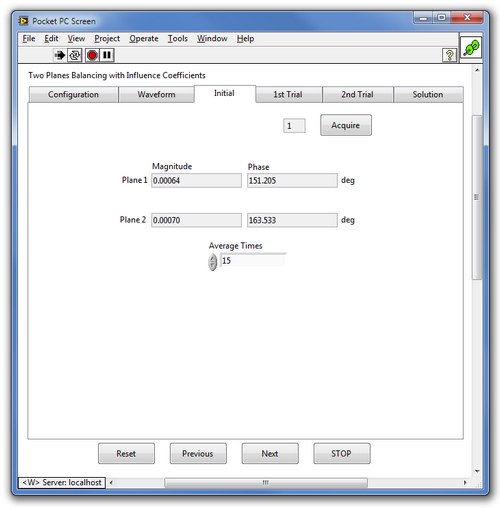Figure 8. Initial recording of the vibration levels of the two accelerometers.

The next step is to place your first trial weight.  A known weight should be added to the first plane at a known angle. These values should be entered in the Weight and Phase controls of the 1st Trial tab in grams and degrees respectively. Once the value are entered, press the Acquire button again to record the values for the first trial.

You also can find a Save button and a Load button on this page. You can use these two buttons to save and load all the environment variables so that you do not have to repeat the measurement if you stop the VI by mistake or any error occurs. You also can use the Save button to save a whole set of measurement results instead of marking them down manually.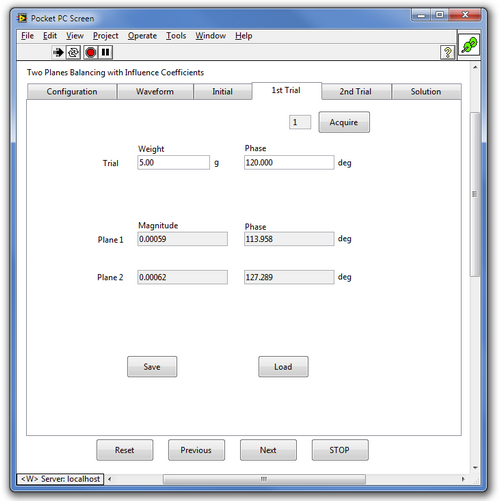Figure 9. The 1st Trial Tab.  The 2nd Trial Tab is identical.

After the 1st trial is complete you should remove the weight from the first plane and place a second trial weight on the second plane at a known angular position.  You can choose to either use the same weight or a different weight.  Enter the weight and position in the controls on the 2nd Trial tab.  Once again, press the Acquire button to record the trial data.  After the 2nd trial is complete, you may remove that weight as well.

The final tab is the Solutions tab.  Once both trials have been completed you can press the Solution button to have your balance weights and angles calculated.  You may then place these weights at the specified angles on the two planes and press the Acquire button again to perform a final, balanced, acquisition.  This should demonstrate a more balanced machine than the initial readings.

### 6. Related Links

NI Machine Condition Monitoring Portal

### 7. Appendix A: Two Plane Balancing Theory

Assume some kind of weight unbalances exist in the two-plane system, you can use the following traditional two-plane vector equations for the initial unbalance response of a linear mechanical system: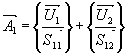(1)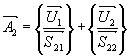(2)

where: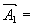Initial Vibration Vector at Bearing 1 (Grs,p-p at Degrees)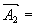Initial Vibration Vector at Bearing 2 (Grs,p-p at Degrees)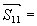Sensitivity Vector at Bearing 1 to Weight at Plane 1 (Grams/Grs,p-p at Degrees)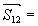Sensitivity Vector at Bearing 1 to Weight at Plane 2 (Grams/Grs,p-p at Degrees)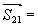Sensitivity Vector at Bearing 2 to Weight at Plane 1 (Grams/Grs,p-p at Degrees)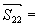Sensitivity Vector at Bearing 2 to Weight at Plane 2 (Grams/Grs,p-p at Degrees)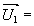Mass Unbalance Vector at Plane 1 (Grams at Degrees)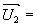Mass Unbalance Vector at Plane 2 (Grams at Degrees)

In order to calculate the influence coefficients of the system, we must add some trial weight at both planes to acquire vibration vectors under different conditions. For a linear system, the addition (or removal) of a calibration weight W1 at plane 1 should vectorially sum with the existing unbalance U1 to produce the following new pair of vector equations: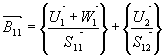(3)(4)

where: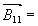Vibration Vector at Bearing 1 with Weight W1 at Plane 1 (Grs,p-p at Degrees)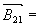Vibration Vector at Bearing 2 with Weight W1 at Plane 1 (Grs,p-p at Degrees)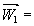Calibration Weight Vector at Plane 1 (Grams at Degrees)

Removal of the calibration weight at balance plane 1, plus another calibration weight W2 at balance plane 2 produces the following pair of vector equations: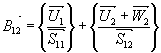(5)(6)

where:Vibration Vector at Bearing 1 with Weight W2 at Plane 2 (Grs,p-p at Degrees)Vibration Vector at Bearing 2 with Weight W2 at Plane 2 (Grs,p-p at Degrees)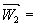Calibration Weight Vector at Plane 2 (Grams at Degrees)

The six equations above contain eight known vector quantities: six vibration vectors and two calibration weights. The calculation procedure initially solves for the four unknown balance sensitivity vectors, and finally the two mass unbalance vectors are calculated. The following expression provides a general solution for balance sensitivity vectors for the solution: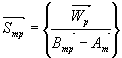(7)

In equation (7), the subscript m specifies the measurement plane, and the subscript p identifies the weight correction plane. Combining the solutions for the four balance sensitivity vectors within the initial equations (1) and (2) yields the following result for mass unbalance at both correction planes: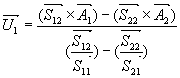(8)(9)

The calculated mass unbalance vectors (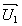and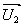) represent the amount of weight that should be used at each balance correction plane. The angles associated with these unbalance vectors represent the angular location of the mass unbalance. Hence, weight can be removed at the calculated angles, or an equivalent weight may be added at the opposite side of the rotor. That is, if weight must be added, the weight addition angle would be equal to the calculated mass unbalance vector angle plus or minus 180º. Although it is generally desirable to remove weight from a rotor during balancing, we only can add balance correction weights to the planes using in this demo. And, calibration weight W2 must be removed from Plane 2 before adding new balance correction weight.

Customer Reviews
3 Reviews | Submit your review

Units  - Feb 1, 2012

Could you please define the (Grs, p-p) unit used for your vibration readings.

vector division?  - Mar 30, 2011

This tutorial uses the cross product of vectors which is well defined, but it also uses the division of vectors which is not. How are the vectors being divided to produce the unbalance vectors? Is it a simple piecewise division?

How To Use This VI  - Mar 9, 2011

Hello Ive tryied to use this VI using a NIUSB6008 DAQ. Plugued a couple of ADXL320 acelerometers to AI0 and AI1 and a digital tachometer on AI2. Now Ive set settings like this: Channel Type is Voltage for al inputs since my accelerometes give information in volts. Range is 5 (volts) for al inputs, sensitivity iv changed a lot from 100 to 1000, IEPE not allowed, coupling DC, and planes an tachometer properly selected. In sample rate and bock size maximum allowable for my DAQ is 3200 each chanel on both paramethers. I run the VI and get readings of vibration of my accelerometers and also speed. Now when I go an do the calibration procedure I get very confusing data on the amplitude levels (what does that level mean) and also the angle is always the same no matter what I do with the rotor to change its weight. So at the end computed results are nonsense, like 50kg for a 20kg rotor??? Can anyone help me out sorthing this problem. I know Im using very cheap sensing instruments but some real aproach must be obtained or am I wrong?? Or perhaps I have a non finished VI?? Regards